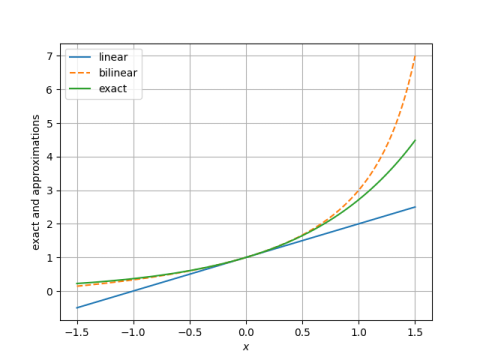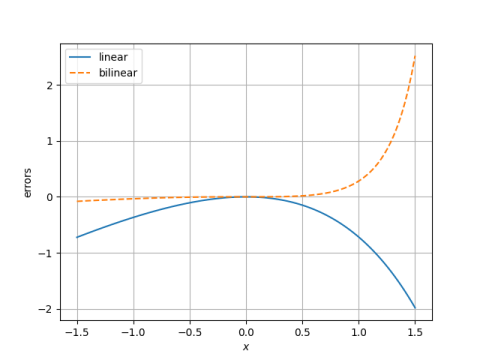# Simple derivation of exponential approximation

I was watching one of Brian Douglas’ videos on control theory (Discrete Control #5) and ran into a simple derivation of an approximation I presented earlier.

Back in April I wrote several post on simple approximations for log, exp, etc. In this post I gave an approximation for the exponential function:The control theory video arrives at the same approximation as follows.As I believe I’ve suggested before here, in a derivation like the one above, where you have mostly equalities and one or two approximations, pay special attention to the approximation steps. The approximation step above uses a first order Taylor approximation in the numerator and denominator.

The plot below shows that the approximation above (the bilinear approximation) is more accurate than doing a single Taylor approximation, approximating exp(x) by 1 + x (linear approximation).Here’s a plot focusing on the error in the bilinear and linear approximations.The bilinear approximation is hard to tell from 0 in the plot above for x up to 0.5.

The derivation above is simple, but why is the result so good? An explanation in terms of Padé approximation is given here.

## 4 thoughts on “Simple derivation of exponential approximation”

1. Not math, but poetically: perhaps alternating “x” signs on the otherwise similar bilinear terms drive error in counter-balancing directions, cancelling out first order error terms.

If so, maybe this could be generalized, and splitting a function into even/odd symmetric parts (x —> -x) before approximation has similar value.

2. Nathan Hannon

A more fair comparison might be to a 2nd order Taylor polynomial, since both have 3 degrees of freedom.

3. Don Fredkin

Here is a “derivation” that is not black magic. Using some plain Tex notation:
The exponential is the solution of the initial value problem
u'(x) = u(x), u(0) = 1.
We can approximate the solution with the trapezoidal formula
u(x) = u(0) + \int_0^x u'(x) dx \approx u(0) + {x \over 2} (u'(0) + u'(x))
so that
u(x) \approx u(0) + {x \over 2} (u(0) + u(x)) = 1 + {x \over 2} (1 + u(x)) .
Solve for u(x) and we have
u(x) \approx {1 + x/2 \over 1 – x/2},
which is the desired approximation.

4. Diethard Michaelis

@Symmetry of Approximation Error.
By (my) intuition the above derivation seemed to enforce
a rather symmetric approximation error.
The absolute error, however, is quite asymmetric.
But both the relative error
(exp(x) – (x+2)/(x-2))/exp(x)
and the absolute log scale error
x – ln((x+2)/(x-2))
are very symmetric and nearly coincide over [-1,1].